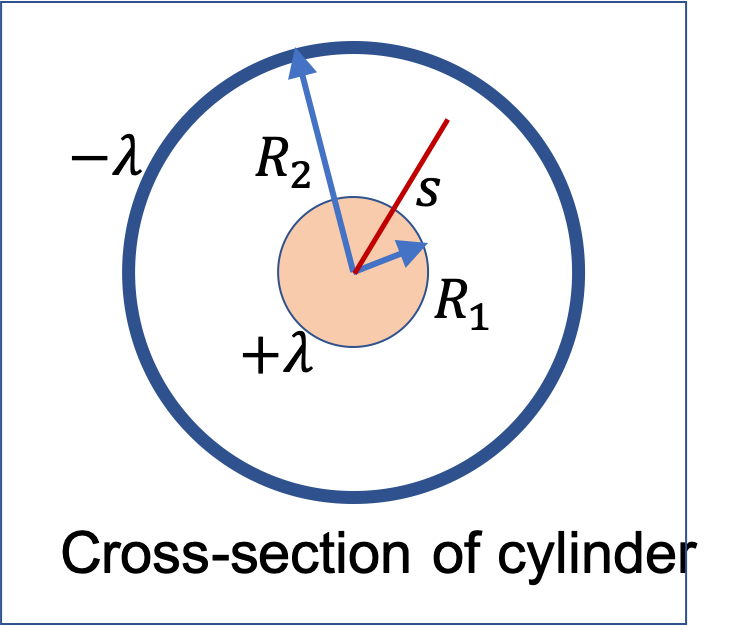## Section34.4Cylindrical Capacitor

In many situations we deal with metal wires that are surrounded by a metal shield.

For instance, in a coaxial cable shown in Figure 34.4.1, the central wire is surrounded by a metallic sheath. This structure shields the current in the central wire from electromagnetic noise. An analysis of coaxial cable requires its capacitance, which we can approximate by the capcitance of cylindrical capacitor.

Consider an infinitely long cylindrical metal wire of outer radius $R_1$ surrounded by metal shell of inner radius $R_2$ as in Figure 34.4.2. For cylindrical symmetry we require the wire to be infintely long, which makes capacitance infinite. Threfore, we will get formula for capacitance per unit length.

We will find below that capacitance per unit length is given by

\begin{equation*} C/L = \dfrac{2\pi\epsilon_0}{\ln\left(R_2/R_1 \right)}, \end{equation*}

if there is nothing between the two conductors, and

\begin{equation*} C/L = \dfrac{2\pi\epsilon_0\epsilon_r}{\ln\left(R_2/R_1 \right)}, \end{equation*}

if there is a material of dielectric constant $\epsilon_r$ filling the space between the conductors.

Derivation of the Capitance Formula

As always, to get capacitance, we will place charges on the two conductors and find the potential difference between them. The ratio of potential difference to the charge will be identified to be the capacitance.

In cylindrical symmetry, we will work with charge per unit length. Let $+\lambda$ be the charge per unit length on the exrernal surface of the inner wire and $-\lambda$ on the inner surface of the outer wire. Let $s$ denote distance to an arbitrary point from the axis. This is cylindrical radial distance.By applying Gauss's law, you can show that the electric field of these charges is

\begin{equation*} E = \begin{cases} 0 \amp s \lt R_1\\ \dfrac{1}{2\pi\epsilon_0}\, \dfrac{\lambda}{s} \amp R_1 \lt r \lt R_2\\ 0 \amp s \gt R_2 \end{cases} \end{equation*}

Integrating this from outer plate to inner plate will give the potential difference. For students with Calculus background, you should do that integral. For othere, use the following answer.

\begin{equation*} V = \phi_+ - \phi_- = \dfrac{\lambda}{2\pi\epsilon_0}\, \ln\left(\dfrac{R_2}{R_1} \right). \end{equation*}

Dividing $\lambda$ by $V\text{,}$ we get capacitance per unit length of a cylindrical capacitor, which we will denote by a compound symbol $C/L\text{,}$ to be

\begin{equation*} C/L = \dfrac{2\pi\epsilon_0}{\ln\left(R_2/R_1 \right)}. \end{equation*}

If the space between the two wires is occupied by an dielectric/insulator of dielectric constant $\epsilon_r\text{,}$ then capacitance is increased by a multiple of $\epsilon_r\text{.}$

\begin{equation*} (C/L)_\text{with dielectric} = \epsilon_r\, C/L = \dfrac{2\pi\epsilon_0\epsilon_r}{\ln\left(R_2/R_1 \right)}. \end{equation*}

An RG59 coaxial cable has inner core of radius $0.28\text{ mm}$ surrounded by plastic of dielectric constant $\epsilon_r = 2.7\text{,}$ which is surrounded by a metal jacket of radius $2.2\text{ mm}\text{.}$

(a) Find the capacitane per unit length for the case in which the space between the wire and metal jacket is vacuum instead of plastic.

(b) Find the capacitane per unit length for the coaxial cable described above.

Hint

Use the formula given in this section.

(a) $115 \text{ pF/m}\text{,}$ (b) $311 \text{ pF/m} \text{.}$

Solution 1 (a)

(a) In the formula for capacitance per unit length the radii enter as ratio, therefore, we do not need to convert units.

\begin{align*} (C/L)_0 \amp = \dfrac{2\pi\epsilon_0}{\ln\left(R_1/R_2 \right)}, \\ \amp = \dfrac{2\pi\times 8.85\times 10^{-12}}{\ln\left(2.2/0.28\right)} = 115 \text{ pF/m}. \end{align*}
Solution 2 (b)

With dielectric between the plates, the capacitance will go up.

\begin{equation*} C/L = \epsilon_r (C/L)_0 = 2.7 \times 115 \text{ pF/m} = 311 \text{ pF/m}. \end{equation*}

A long glass cylindrical shell made of $4\text{ mm}$ thick Pyrex is used to make a capacitor. The inside and outside of the cylindrical shell is lined with a thin aluminum foil. What is the capacitance per unit length if the inside radius is $5\text{ cm}\text{?}$

Data: $\epsilon_{r,\text{ glass} }= 4.7$

Hint

Use $C/L=\epsilon_r (C/L)_0$

$3 \text{ nF/m}\text{.}$

Solution

The capacitance per unit length for a cylindrical capacitor of radii $R_1$ and $R_2$ of the inner and outer plates with vacuum between them is given by

\begin{equation*} (C/L)_0 = \dfrac{2\pi\epsilon_0}{\ln(R_2/R_1)}, \end{equation*}

With pyrex between the plates this will be multiplied by the dielectric constant $\epsilon_r$ of pyrex.

\begin{equation*} C/L = \epsilon_r\: \dfrac{2\pi\epsilon_0}{\ln(R_2/R_1)}. \end{equation*}

Putting in the given dimensions we obtain

\begin{equation*} C/L = 4.7\: \dfrac{2\pi\times 8.85\times 10^{-12}}{\ln(5.4/5)} = 3\times 10^{-9}\:\text{F/m}. \end{equation*}